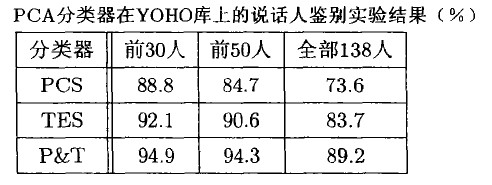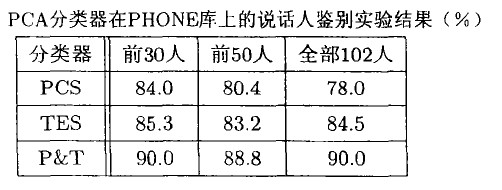## 1.说话人分类常用算法

(1)基于概率分布的高复杂度的精确分类算法，如高斯混合模型（Gaussian Mixture Model，GMM）、隐马尔科夫 模型（Hidden Markov Model，HMM）和支持向量机（Support Vector Machine，SVM）模型；
(2)基于模板的低复杂度的分类算法，如向量量化方法（Vector Quantization，VQ）。

Wu Junwen，Zhang Xuegong提出了一种基于PCA的分类器（即主成分子空间（Principal Component Subspace，PCS）分类器）， 作为一种线性分类器，其复杂度低于以上第一类算法，又表现出很好的分类能力。另外，我们还可以根据截断误差子空间来构造分类器， 称为TES（Truncation Error Subspace）分类器。这两种分类器反映了主成分分析方法的两个方面，结合两种分类器可得到混合分类器， 称其为P&T分类器。

## 2.主成分分析的分类依据

PCS分类器的基本思路是，对于一个待分类样本$X$，将它向数据集中每个说话人模型（即PCS）投影，即 计算$U{k}^{T}(X-\mu {k})$，其中$U{k}$为数据集中第$k$个说话人的模型，$\mu {k})$为每个模型的中心向量，定义投影 量所保留的原向量的方差为：

$f(k)=\parallel U{k}^{T}(X-\mu {k})\parallel ^{2}，k=1，2，…，n$.

$x \in model${ $k|max(f(k)),1 < k \leq n$ }.

TES分类器与PCS分类器类似，只是说话人模型是用TES来表示的，对应的说话人模型相似度的度量标准为：

$g(k)=\parallel V{k}^{T}(X-\mu {k})\parallel ^{2}, k=1, 2, ..., n$.

$x\in model$ { $k|min(g(k)),1 < k \leq n$ }.

P&T分类器是将以上两种分类器结合起来进行决策，最简单的结合方法是线性组合，可以设PCS分类器的 权重为$\lambda （0\leq \lambda \leq 1）$，而TES分类器的权重为1-λ，则衡量说话人模型相似度的标准为：
$h(k)=\lambda f(k) - (1-\lambda)g(k), k=1, 2, ..., n$.

$x\in model$ { $k|min(h(k)),1 < k \leq n$ }.

## 3.主成分分类器应用于说话人识别

(1)训练说话人模型；
(2)对测试音进行鉴别。

$F(k) = \sum{n=1}^{N} \parallel U{k}^{T}(X(n)-\mu _{k}) \parallel ^{2}, k = 1,2,...,M$.

## 4.实验结果## 5.复杂度分析

P&T分类器的复杂度

$PTtrain = Nd+Nd+ddN+4/3d^{3}+O(d^{2})+O(dlogd) = O(Nd^{2})+O(d^{3})$ 模型测试阶段： $PTtest=MNtestPd+MNP+O(1) =O(MNtestP*d)$

## 参考文献

《说话人识别模型与方法》吴朝辉 杨迎春 著 清华大学出版社
基于PCA与LDA的说话人识别研究 章万峰 浙江大学硕士论文